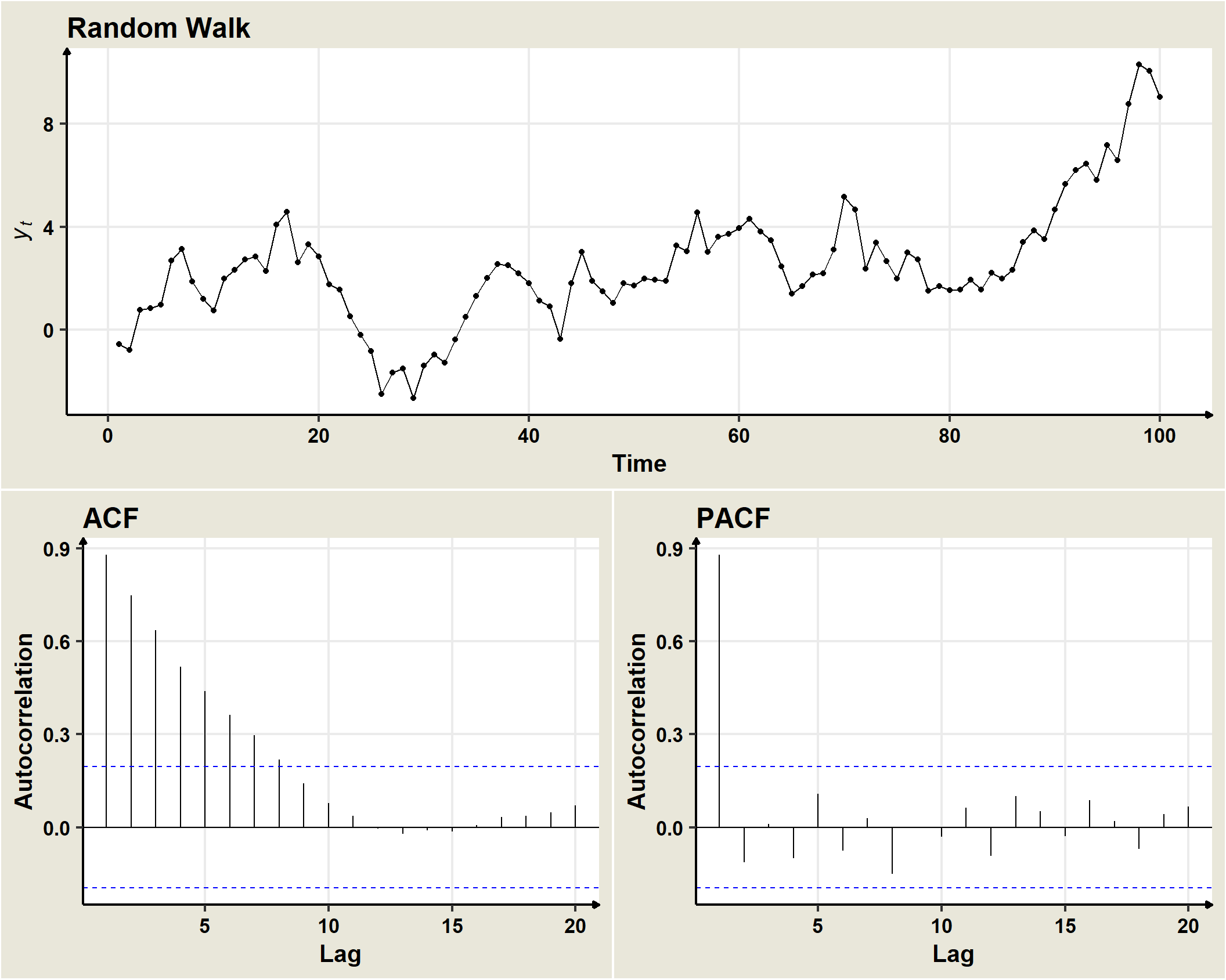## 19.2 Random walk

A non stationary stochastic process $$(Y_t)$$ is a random walk when:

$Y_t=Y_{t-1} + \omega_t$• A random walk is a stochastic process whose first difference is a white noise, i.e.

$Y_t - Y_{t-1}=\omega_t$ * Sometimes an additional parameter is included:

$Y_t = \mu + Y_{t-1} + \omega_t$

• A random walk can be written as:

$\nabla Y_t = \mu + \omega_t,$

so that a non-stationary stochastic process $$(Y_t)$$ is a random walk when its regular difference of order 1 $$\left(Y_{t} \right ) \equiv \left ( Y_{t}-Y_{t-1} \right )$$ is a stationary process.